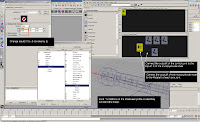## Monday, May 21, 20073 BONE BICEP SETUP FOR TWIST MINIMIZING

Here is an arm setup which uses a control joint to drive 3 joints from the shoulder to the elbow using a multiply/divide node. This will lock the shoulder twisting and disperse it through the bicep.

1. Select joints you wish to link the roll (x) to - shoulderControlJoint, bicepJointA and bicepJointB
2. Open the hypershade
3. Click on the "show upstream and downstream connections" icon to show the shoulderControlJoint bicepJointA and bicepJointB in the hypershade.
4. Create a "multiply Divide" node (Create>General Utilities>Multiply Divide )
5. Change "input2 X" on the multiply/Divide from 1 to .5 (since we are halving and the node is set to multiply)
6. Now middle click drag and connect the "Rotate X" of the shoulderControlJoint to the "Input1 X" of the multiply/Divide node. (This is the value that is going to be halved.)
7. Connect the "Output X" of the multiplyDivide to the "Rotate X" of each bicep bone. (This is the value after it has been halved.)